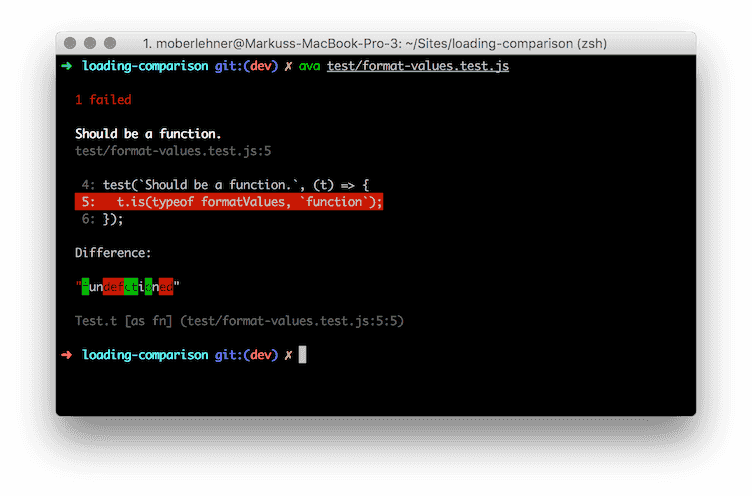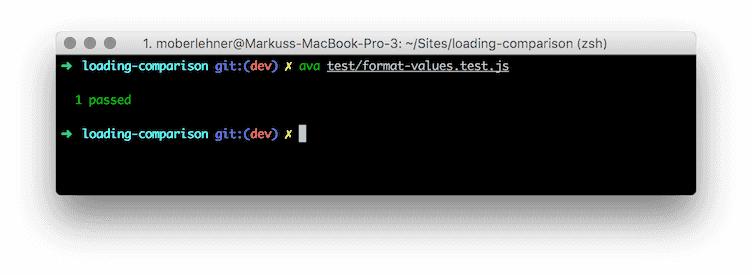# Test Driven Development with JavaScript Using ava and Sinon.JS

For a long time testing and test-driven development (TDD) was a magical thing for me. I didn’t really know what it meant and it seemed to be something only “real” developers can do correctly. Many developers suffer from imposter syndrome and so did (sometimes even today do) I and I was too scared to get into this magical thing called TDD.

Two or three years ago I started to work on some open source projects and I needed a way to stop things from breaking because of changes made to the codebase. I bit the bullet and started to write tests for my projects. I did everything wrong what you can do wrong, but tagging a new release knowing everything still works as expected is just a great feeling. It was amazing

Up until this day I’m still learning how to do this testing thing correctly and most of the time I still write my tests after I wrote the code (so I actually don’t do TDD).

## Writing testable code

For the longest time I did quite some things wrong when writing tests for my projects. I didn’t get that you have to actually write testable code in order to write good tests for it.

Let’s take the following code from my most recent project (loading-comparison) as an example.

``````const chalk = require(`chalk`);

module.exports = function formatValues(values) {
const minValue = Math.min(...values);
return values.map((value) => {
if (value === minValue) return chalk.bold.green(value);
return value;
});
};``````

Whats wrong with this code when it comes to testability? The `formatValues` function depends on functions in the global scope (`chalk` and `Math`). Why is this bad? When testing this function we are limited in what we can test. In fact we can only test the output of the function. If we change something and the test fails, the only thing we know is that something is wrong but not exactly what. We are not able to determine if the `Math` or the `chalk` functions are called with the correct values or if they are called at all.

## Refactoring with TDD

So let’s rebuild the `formatValues` function using the TDD approach.

### Setting up ava

Although I’m used to mocha, sometimes I want to try new stuff and I choose ava to experiment with. Why ava? It is new and has 8.700 stars on GitHub, so it must be good, right?

Let’s install ava and Sinon.JS and add a new `test` script to our `package.json` file to get started.

``npm add -D ava sinon``
``````{
"scripts": {
"test": "ava"
}
}``````

### Writing our first (failing) test

Because we are real developers who only do real TDD, we start with creating our first test before we are writing any actual code.

``````// test/format-values.test.js
import sinon from 'sinon';
import test from 'ava';

test(`Should be a function.`, (t) => {
t.is(typeof formatValues, `function`);
});``````

This might seem like a silly test, obviously we need a function and obviously there will be a function `formatValues` once we define it. So yeah, you may not write tests for the existence of the function itself in your codebase, but I like the idea of having tests documenting the code. What this test is saying is: “In this codebase, there is a function called `formatValues`”.

Although it might seem superfluous, it is a good habit to always run your tests (and see them fail) once you wrote a new test. That way you might catch an error in your test – if you wrote a new test and the test is not failing, something is wrong with your test (assuming you are doing TDD and write your tests before the actual code).### Make our first test succeed

Now we have a failing test – let’s make it succeed.

``````// lib/format-values.js
function formatValues({}) {}

module.exports = function formatValuesFactory(dependencies) {
return formatValues.bind(null, dependencies);
};``````

Whats going on here? We are creating an empty function which takes an object literal as the first parameter (using the ES6 destructuring assignment syntax). And we define and export a factory function to bind the dependencies (which will come later) to our function and return it. It will become clearer what we are doing here in the next step, trust me.

Usually you shouldn’t have to change your test after writing your code, but in this special case (because we created a new file) we have to update our test to import and use this new file.

``````import formatValuesFactory from '../lib/format-values';

test(`Should be a function.`, (t) => {
const formatValues = formatValuesFactory({});

t.is(typeof formatValues, `function`);
});``````

Let’s run the test and see it succeed.Our test succeeds and that’s great, but our code doesn’t do much. In fact, it does nothing at all, so let’s change that. But first, we add a new test, off course.

``````test(`Should call Math.min().`, (t) => {
const Math = { min: sinon.spy() };
const values = [1, 2];
const formatValues = formatValuesFactory({ Math });

formatValues(values);
t.true(Math.min.calledWith(...values));
});``````

What do we actually want to achieve with our little `formatValues` function? The `formatValues` function should take an array of values and return a new array with the min values of the array highlighted as bold and green when outputted in the terminal.

The first step on the journey to achieve this goal is to find the min values in our array. The native `Math.min()` function does exactly that, except that it doesn’t take an array as parameter but all the values as multiple parameters. Luckily we can use the ES6 spread operator (`...`) to create multiple parameters from a single array.

We want our function to call `Math.min()` with the parameters from an array. We use an object literal and `sinon.spy()` to create a fake `Math.min()` function. This enables us to spy on the fake function and detect if it was actually called.

The test is ready, now we can make it succeed by updating our function.

``````function formatValues({ Math }, values) {
Math.min(...values);
}``````

Now we know what our min values are. Let’s paint them with chalk. But not so fast, first things first: the test.

``````test(`Should format the min values of an array.`, (t) => {
const chalk = {
bold: {
green: sinon.spy(),
},
};
const Math = { min: sinon.stub().returns(1) };
const values = [1, 2, 3];
const formatValues = formatValuesFactory({ Math, chalk });

formatValues(values);
t.true(chalk.bold.green.calledWith(1));
});``````

We now have to fake both, `chalk` and `Math`. For `chalk.bold.green()` we use `sinon.spy()` again. For `Math.min()` we use a `stub`. Because we are smart people, we already know that the min value of `[1, 2, 3]` is `1` so we make `Math.min()` return `1`. What we are testing is, if `chalk.bold.green()` is called with the determined min value of `1`.

After checking if our new test fails as expected, we update `formatValues` to do what our test says it should do.

``````function formatValues({ Math, chalk }, values) {
const minValue = Math.min(...values);
values.map((value) => {
if (value === minValue) return chalk.bold.green(value);
});
}``````

### Testing the return value

At this point we are almost done. The last thing we want our function to do is, to return the new array with the highlighted values.

``````test(`Should return an array with the min values highlighted.`, (t) => {
const chalk = {
bold: {
green: () => `highlighted`,
},
};
const Math = { min: sinon.stub().returns(1) };
const values = [1, 2, 3, 1];
const expectedResult = [`highlighted`, 2, 3, `highlighted`];
const formatValues = formatValuesFactory({ chalk, Math });
const formattedValues = formatValues(values);

t.deepEqual(formattedValues, expectedResult);
});``````

This time we make `chalk.bold.green()` return `highlighted` this should replace the min values of our array with `highlighted` and we can check it by testing if the array returned from `formatValues` equals the values in our `expectedResult` array.

Currently we do not return anything in the `formatValues` function, let’s change that and make our final test succeed.

``````function formatValues({ Math, chalk }, values) {
const minValue = Math.min(...values);
return values.map((value) => {
if (value === minValue) return chalk.bold.green(value);
return value;
});
}``````

Finally our function does what we expect it to do, all tests succeed and we feel great!

## Final thoughts

You may have noticed, that `formatValuesFactory()` is called for every test case. In your “real” codebase you won’t do that. The factory function is called only once or at most once in every controller or entry file or whatever, in your project. The reason why we do this in the test script is, because we want a clean environment for every test case. If we would call the factory function once at the beginning of the test file, and use the same returned function for every test case, there might be side effects caused by the way the (factory) function works. Because of the pure nature of our `formatValues` function, it doesn’t matter, we could use the same instance without fearing side effects but it is a good habit to always use new instances for every test case.

TDD is fun but there are many things you can do wrong. There are many things I did and still do wrong, but the worst thing you can do is to not test at all.

## Do you want to learn how to build advanced Vue.js applications?

Register for the Newsletter of my upcoming book: Advanced Vue.js Application Architecture.

## Do you enjoy reading my blog?

You can buy me a ☕️ on Ko-fi!